Plots for model-based clustering results, such as BIC, classification, uncertainty and density.

# S3 method for Mclust
plot(x, what = c("BIC", "classification", "uncertainty", "density"),
dimens = NULL, xlab = NULL, ylab = NULL,
addEllipses = TRUE, main = FALSE, ...)

Arguments

x

Output from Mclust.

what

A string specifying the type of graph requested. Available choices are:

"BIC"

plot of BIC values used for choosing the number of clusters.

"classification" =

a plot showing the clustering. For data in more than two dimensions a pairs plot is produced, followed by a coordinate projection plot using specified dimens. Ellipses corresponding to covariances of mixture components are also drawn if addEllipses = TRUE.

"uncertainty"

a plot of classification uncertainty. For data in more than two dimensions a coordinate projection plot is drawn using specified dimens.

"density"

a plot of estimated density. For data in more than two dimensions a matrix of contours for coordinate projection plot is drawn using specified dimens.

If not specified, in interactive sessions a menu of choices is proposed.

dimens

A vector of integers specifying the dimensions of the coordinate projections in case of "classification", "uncertainty", or "density" plots.

xlab, ylab

Optional labels for the x-axis and the y-axis.

A logical indicating whether or not to add ellipses with axes corresponding to the within-cluster covariances in case of "classification" or "uncertainty" plots.

main

A logical or NULL indicating whether or not to add a title to the plot identifying the type of plot drawn.

...

Other graphics parameters.

Details

For more flexibility in plotting, use mclust1Dplot, mclust2Dplot, surfacePlot, coordProj, or randProj.

Examples

# \donttest{
precipMclust <- Mclust(precip)
plot(precipMclust)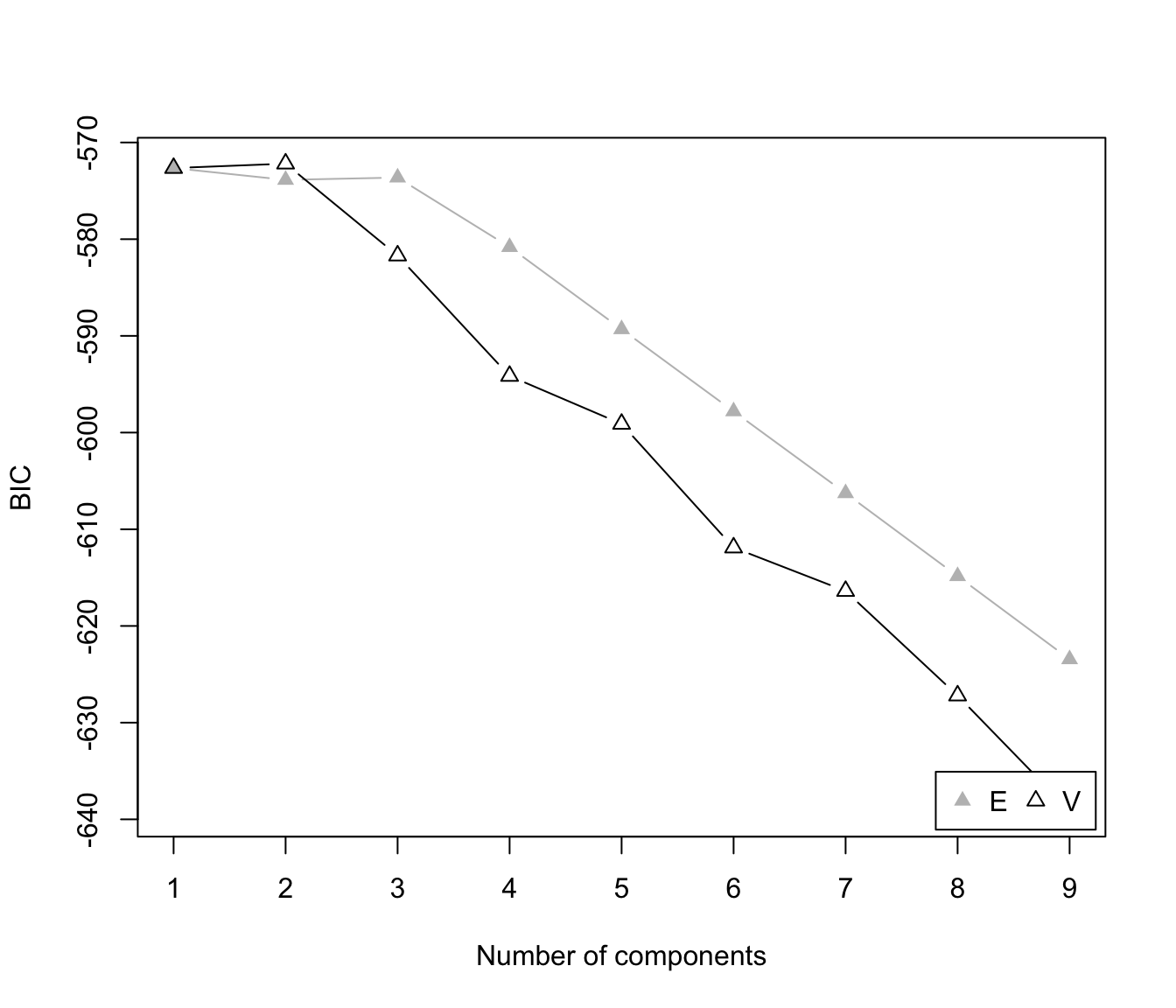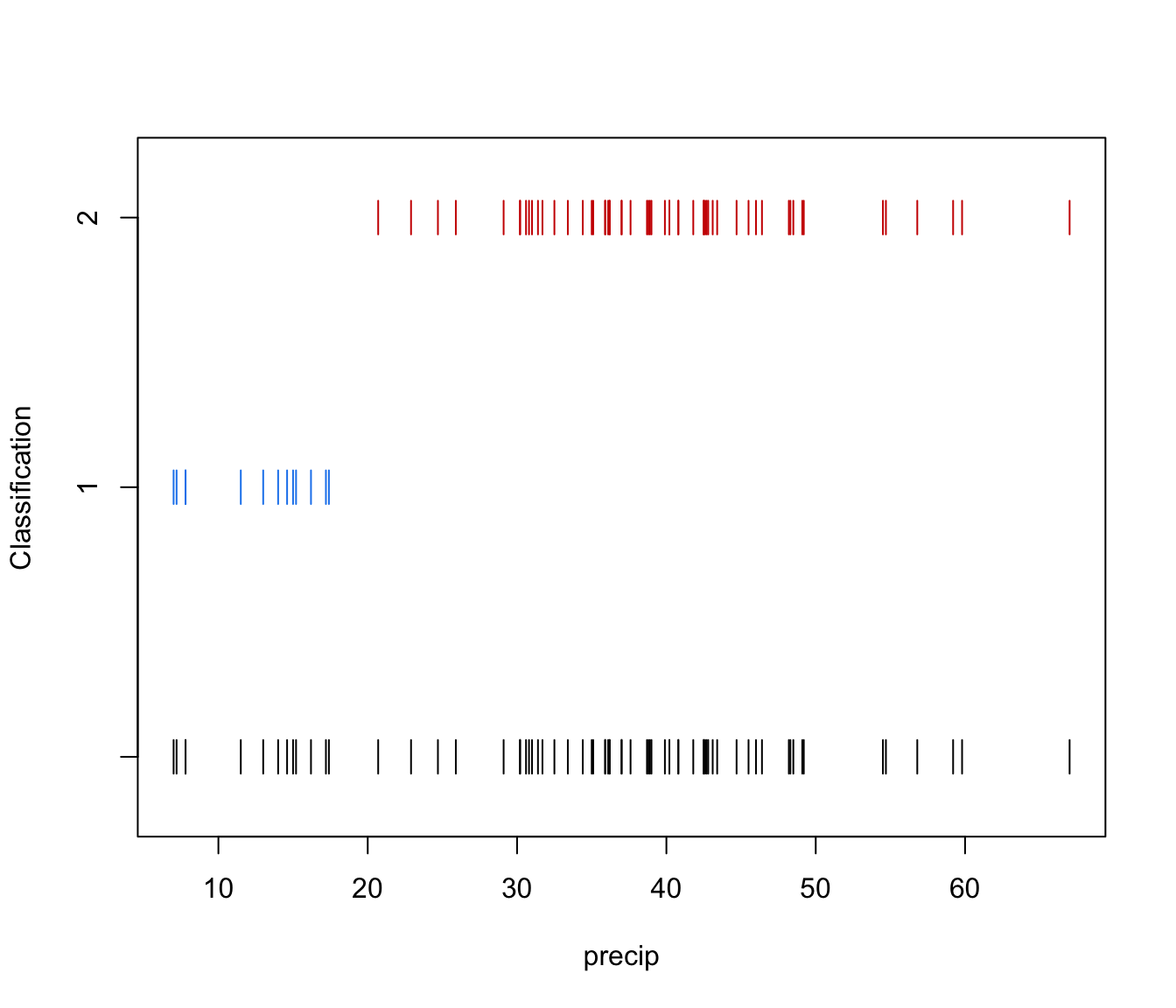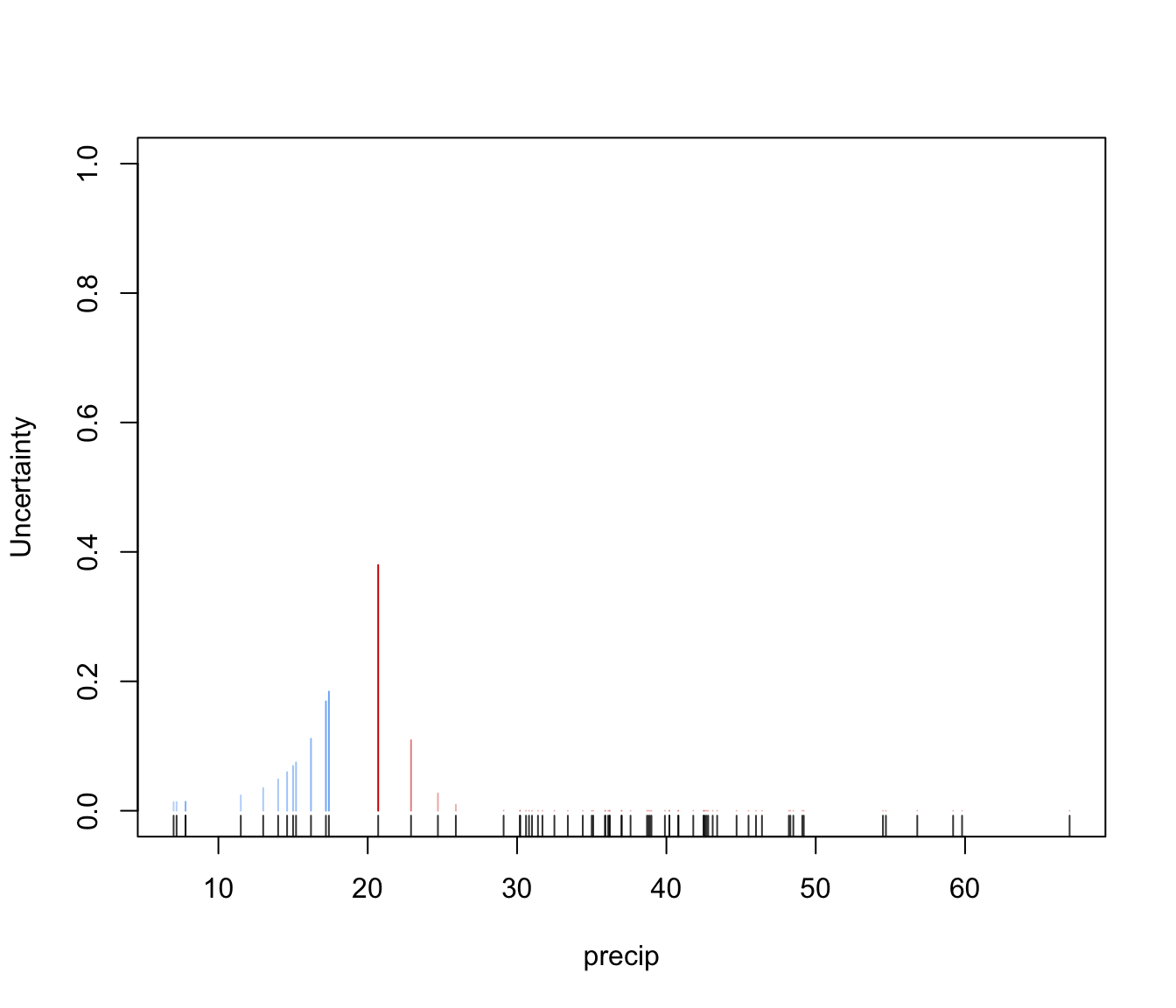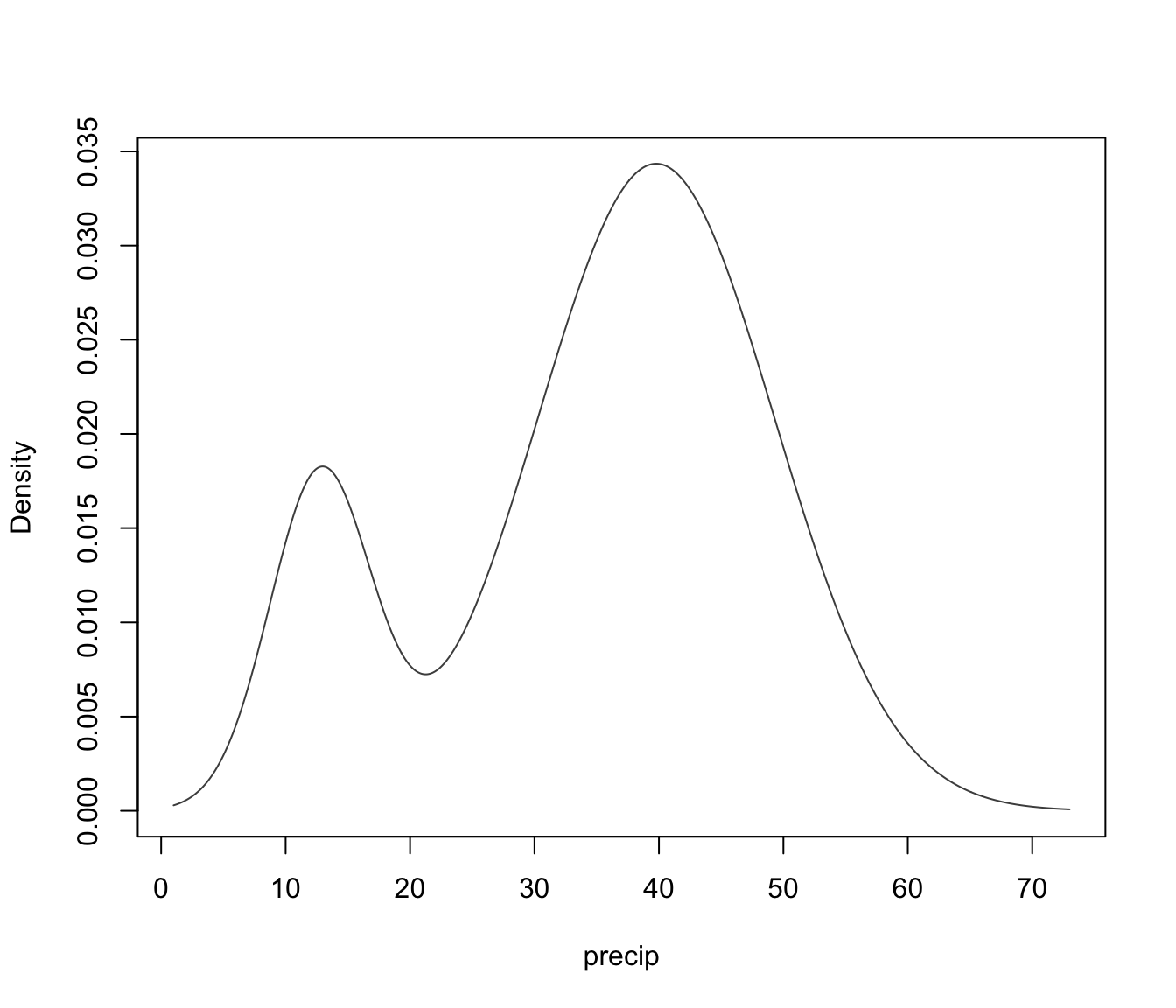faithfulMclust <- Mclust(faithful)
plot(faithfulMclust)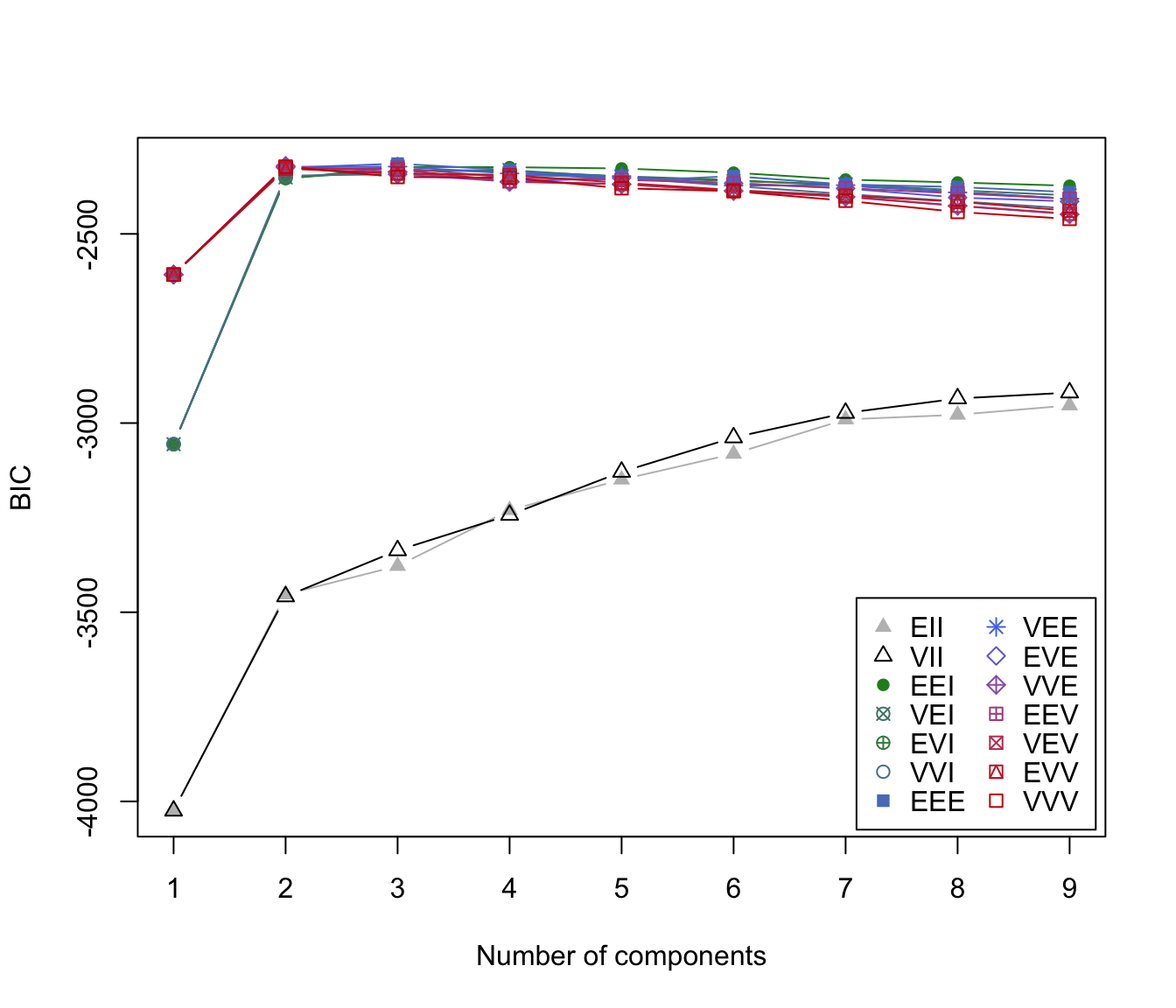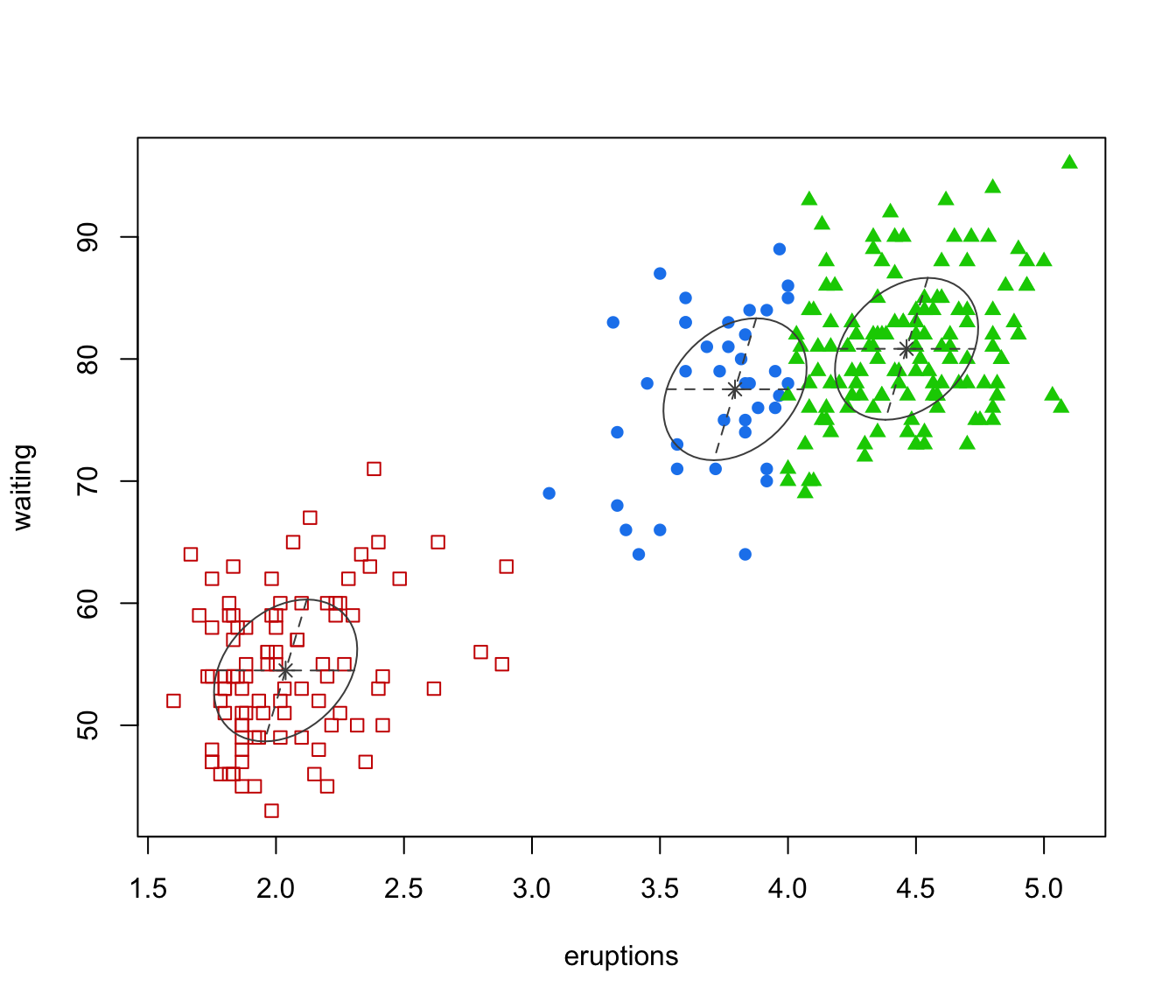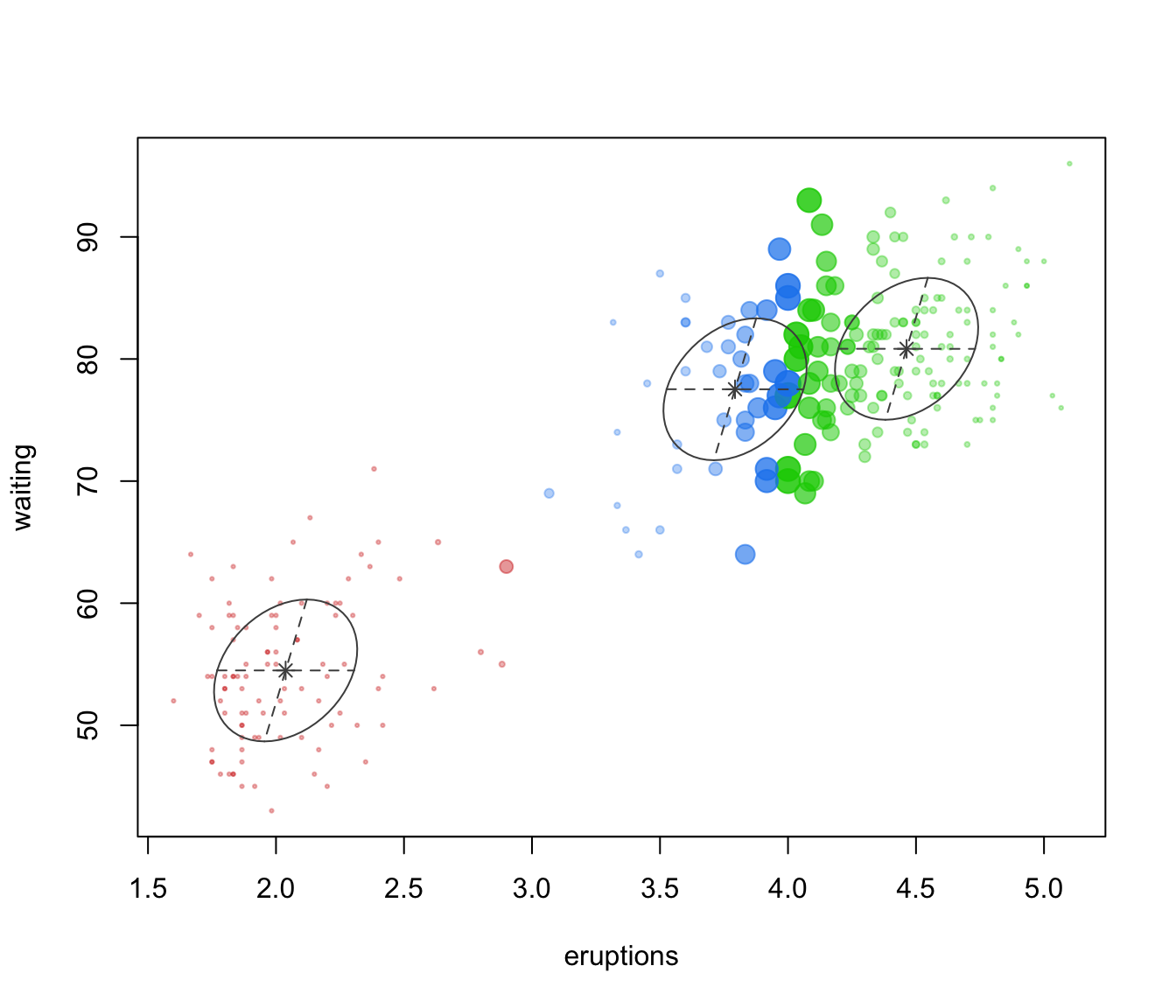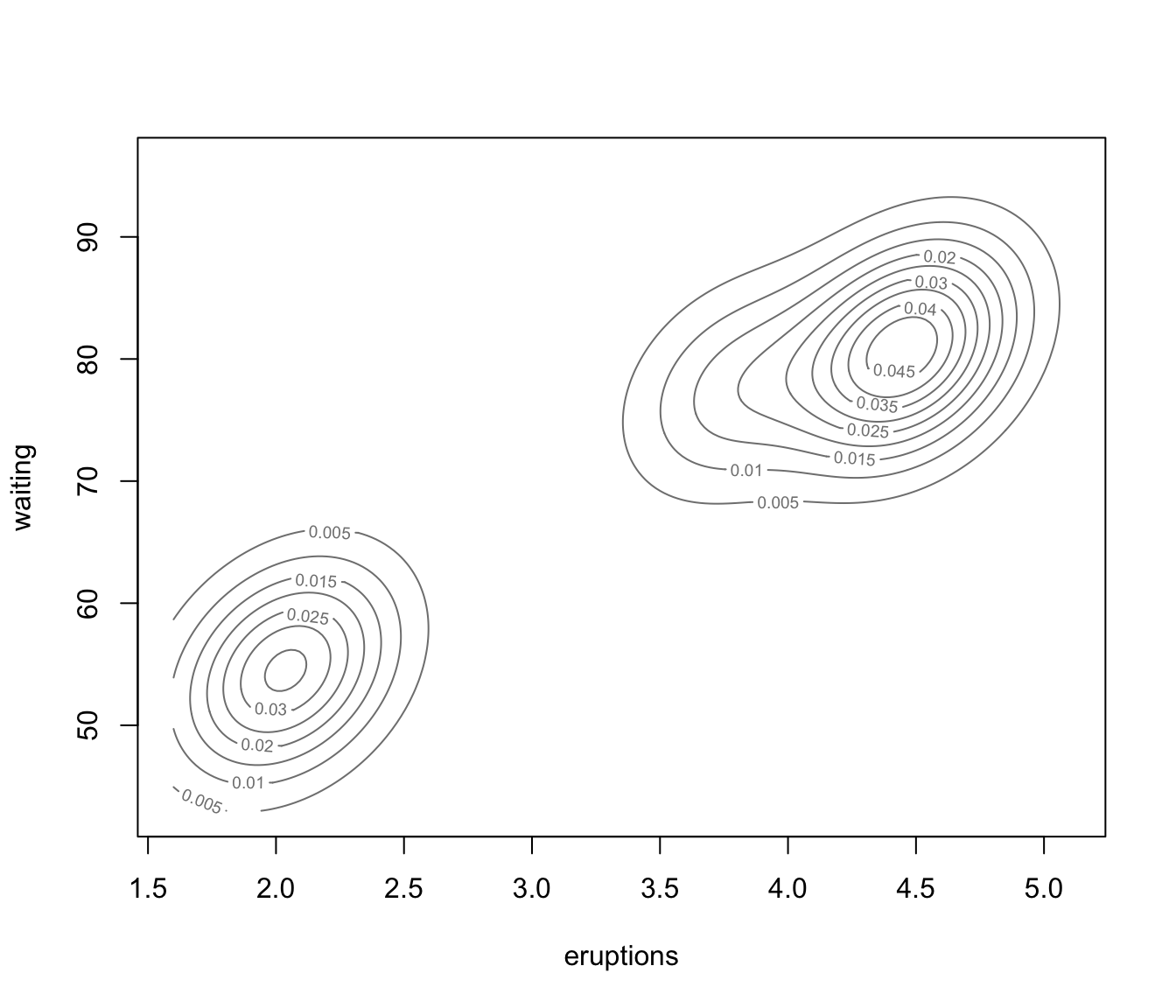irisMclust <- Mclust(iris[,-5])
plot(irisMclust)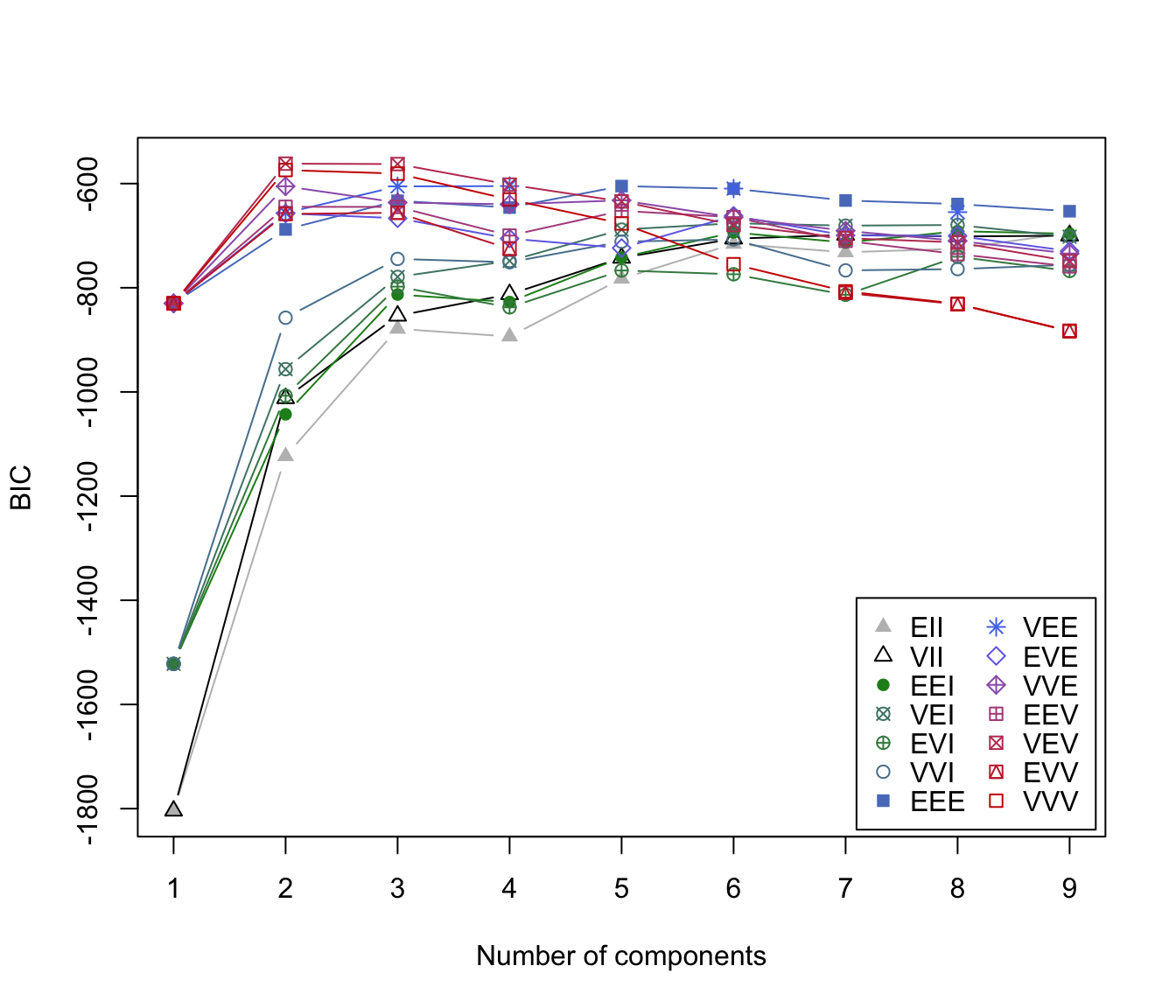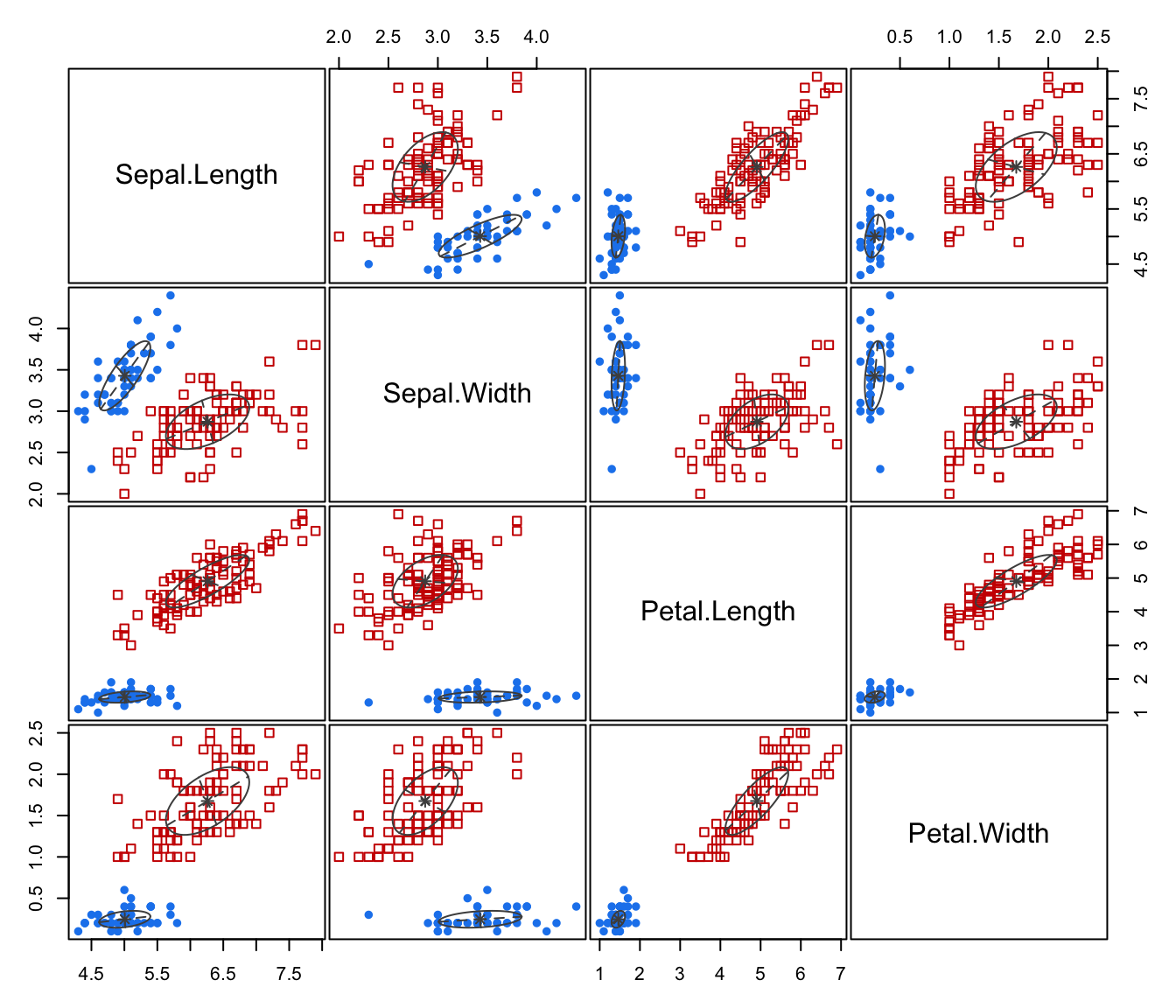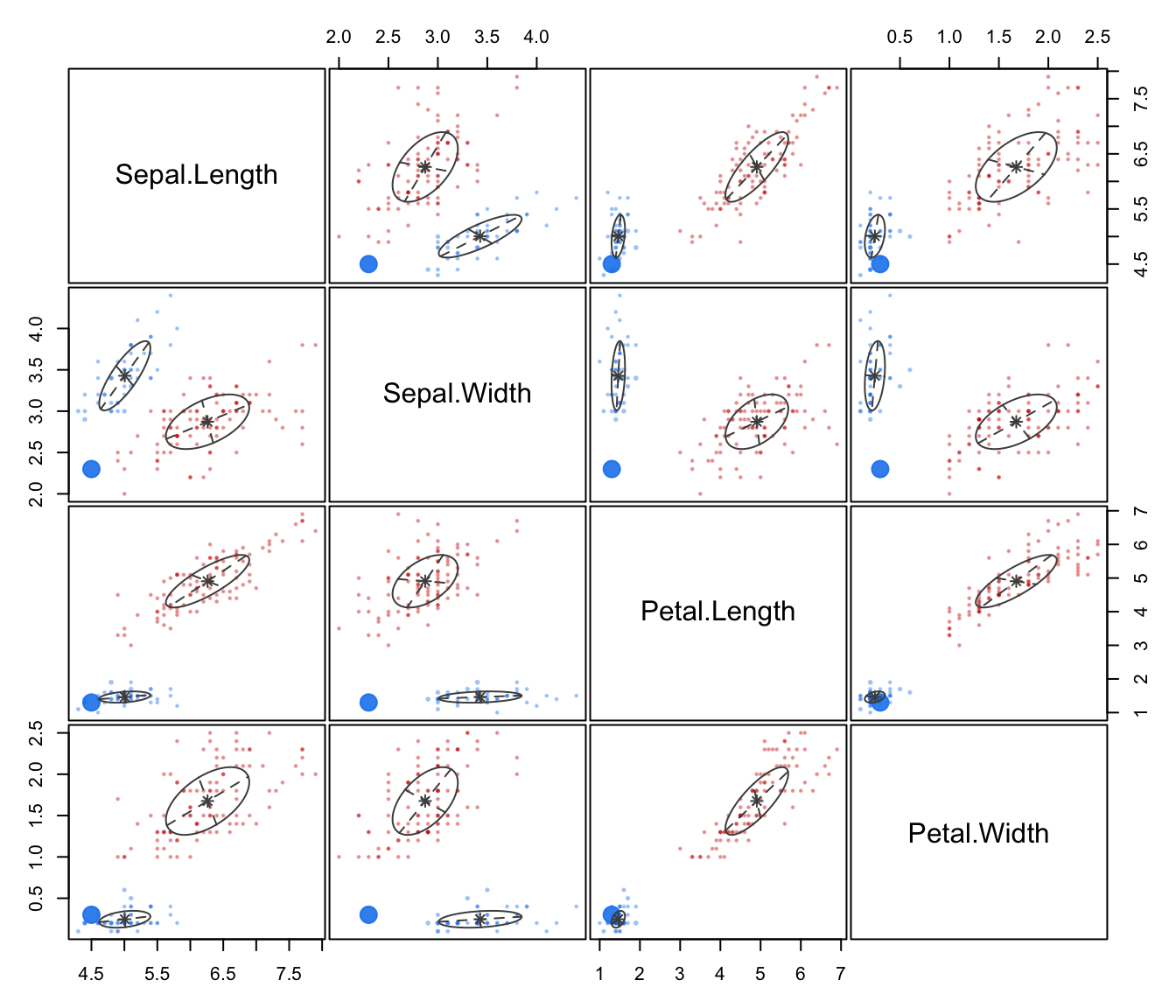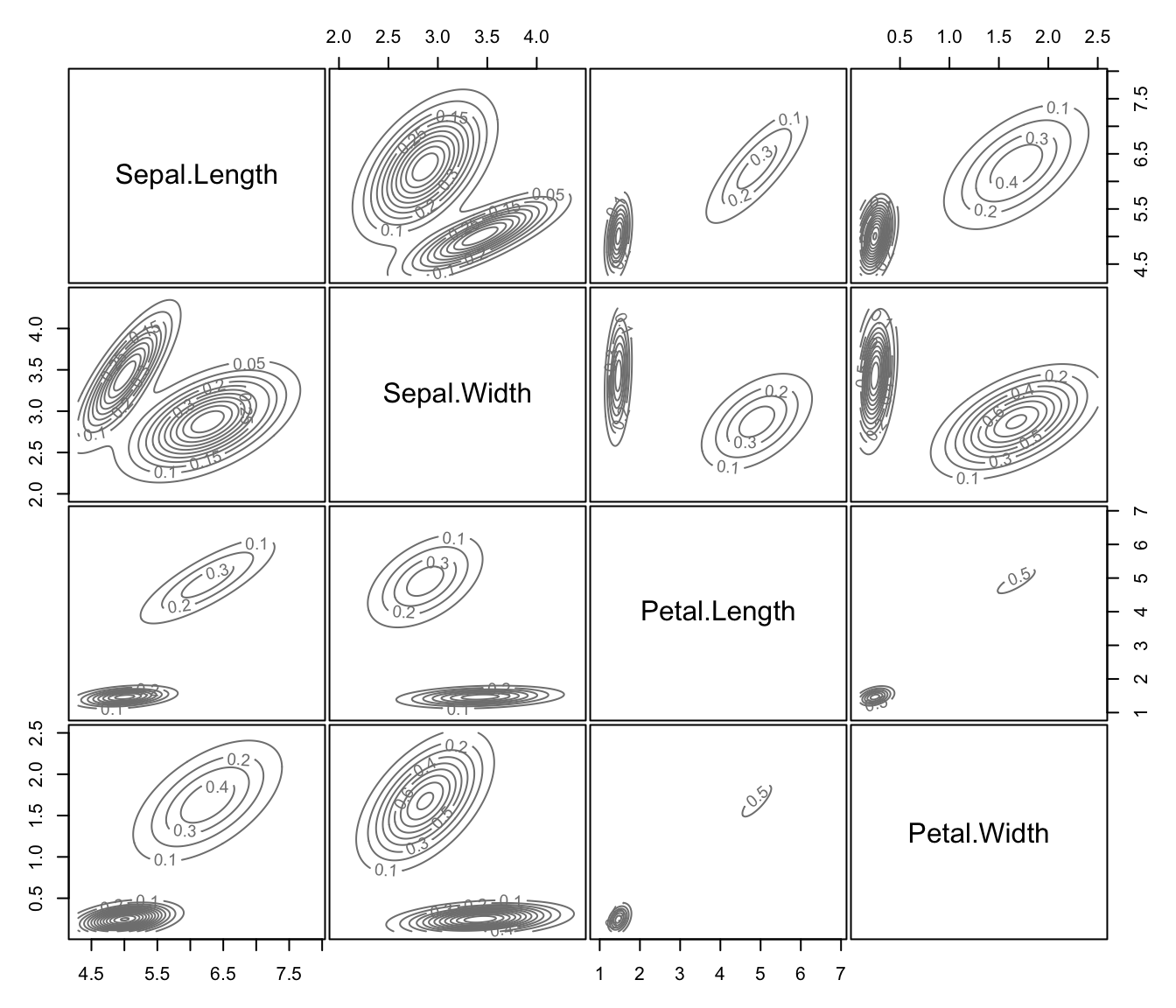# }